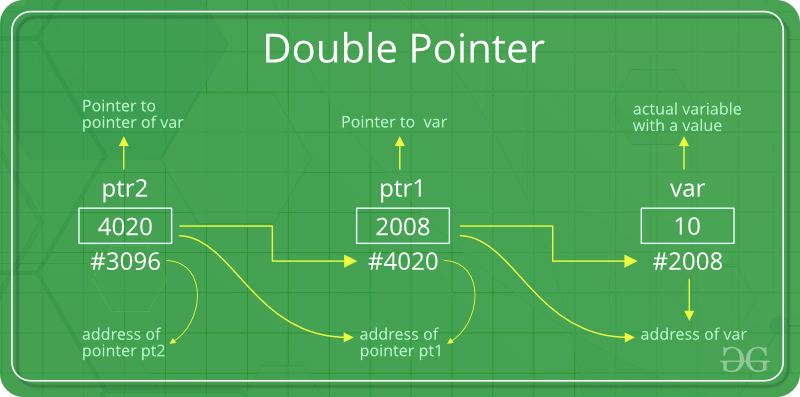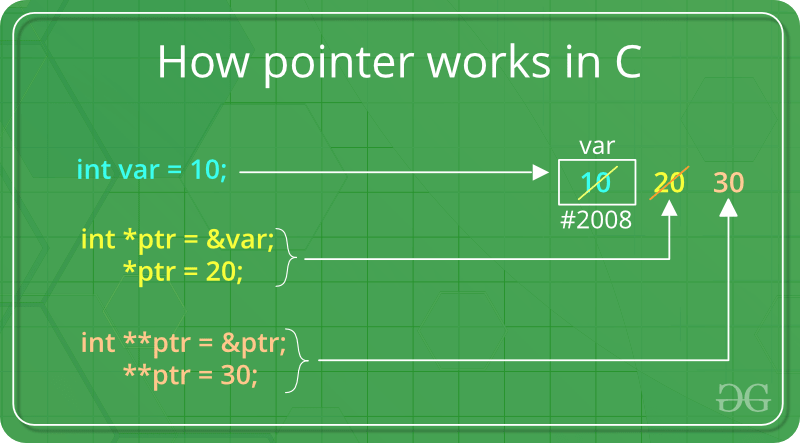Open in App
Not now

# C – Pointer to Pointer (Double Pointer)

• Difficulty Level : Easy
• Last Updated : 25 Oct, 2022

Prerequisite: Pointers in C and C++

We already know that a pointer points to a location in memory and is thus used to store the address of variables. So, when we define a pointer to a pointer. The first pointer is used to store the address of the variable. And the second pointer is used to store the address of the first pointer. That is why they are also known as double-pointers. We can use a pointer to a pointer to change the values of normal pointers or create a variable-sized 2-D array. A double pointer occupies the same amount of space in the memory stack as a normal pointer.

### How to Declare a Pointer to a Pointer in C?

Declaring Pointer to Pointer is similar to declaring a pointer in C. The difference is we have to place an additional ‘*’ before the name of the pointer.

Syntax:

`data_type_of_pointer **name_of_variable = & normal_pointer_variable;`

Example:

```int val = 5;
int *ptr = &val; // storing address of val to pointer ptr.
int **d_ptr = &ptr; // pointer to a pointer declared
// which is pointing to an integer.```

The below diagram explains the concept of Double Pointers:The above diagram shows the memory representation of a pointer to a pointer. The first pointer ptr1 stores the address of the variable and the second pointer ptr2 stores the address of the first pointer.Let us understand this more clearly with the help of the below program:

## C

 `// C program to demonstrate pointer to pointer``#include `` ` `int` `main()``{``    ``int` `var = 789;`` ` `    ``// pointer for var``    ``int``* ptr2;`` ` `    ``// double pointer for ptr2``    ``int``** ptr1;`` ` `    ``// storing address of var in ptr2``    ``ptr2 = &var;`` ` `    ``// Storing address of ptr2 in ptr1``    ``ptr1 = &ptr2;`` ` `    ``// Displaying value of var using``    ``// both single and double pointers``    ``printf``(``"Value of var = %d\n"``, var);``    ``printf``(``"Value of var using single pointer = %d\n"``, *ptr2);``    ``printf``(``"Value of var using double pointer = %d\n"``, **ptr1);`` ` `    ``return` `0;``}`

Output

```Value of var = 789
Value of var using single pointer = 789
Value of var using double pointer = 789```

### What will be the size of a pointer to a pointer in C?

In the C programming language double pointer behave similarly to a normal pointer in C. So, the size of the double-pointer variable and the size of the normal pointer variable is always equal.

## C

 `// C program to demonstrate sizeof normal and double pointer.``#include `` ` `int` `main()``{`` ` `    ``int` `a = 5;``    ``int``* ptr = &a;``    ``int``** d_ptr = &ptr;`` ` `    ``printf``(``" Size of normal Pointer: %d \n"``, ``sizeof``(ptr));``    ``printf``(``" Size of Double Pointer: %d \n"``, ``sizeof``(d_ptr));`` ` `    ``return` `0;``}`

Output

``` Size of normal Pointer: 8
Size of Double Pointer: 8 ```

Note: The output of the above code also depends on the type of machine which is being used. The size of a pointer is not fixed in the C programming language and it totally depends on other factors like CPU architecture and OS used. Usually, for a 64-bit Operating System, the size will be 8 bytes and for a 32-bit Operating system, the size will be 4 bytes.

### What if we want to change the value of a double pointer?

In this case, we can use a triple pointer, which will be a pointer to a pointer to a pointer i.e, int ***t_ptr. And similarly to change the value of a triple pointer we can use a pointer to a pointer to a pointer to a pointer. In other words, we can say that to change the value of a ” level – x ” variable we can use a ” level – x+1 ” pointer. And this concept can be extended further.

Must Read – Function Pointer in C